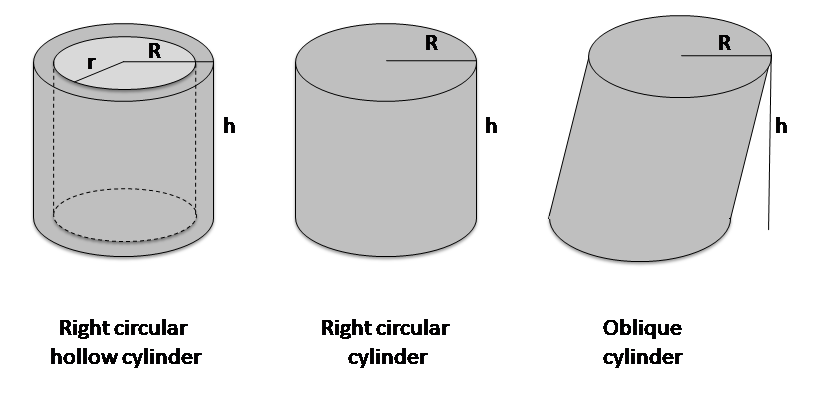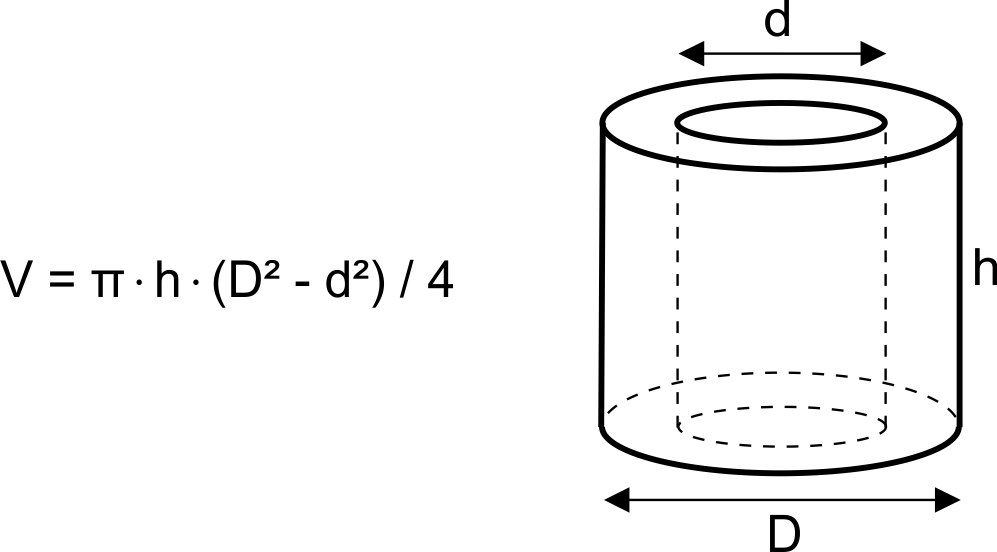# Cylinder Volume Calculator

Created by Hanna Pamuła, PhD candidate
Reviewed by Bogna Szyk and Jack Bowater
Last updated: Sep 06, 2022

Our cylinder volume calculator enables calculating the volume of that solid. Whether you want to figure out how much water fits in a can, coffee in your favorite mug, or even the volume of a drinking straw – you're in the right place. The other option is calculating the volume of a cylindrical shell (hollow cylinder).

## How to calculate volume of a cylinder?

Let's start from the beginning – what is a cylinder? It's a solid bounded by a cylindrical surface and two parallel planes. We can imagine it as a solid physical tin having lids on top and bottom. To calculate its volume, we need to know two parameters – the radius (or diameter) and height:

 cylinder volume = π × cylinder radius² × cylinder height

The cylinder volume calculator helps in finding the volume of right, hollow and oblique cylinders:## Volume of a hollow cylinder

The hollow cylinder, also called the cylindrical shell, is a three-dimensional region bounded by two right circular cylinders having the same axis and two parallel annular bases perpendicular to the cylinders' common axis.

It's easier to understand that definition by imagining, e.g., a drinking straw or a pipe – the hollow cylinder is this plastic, metal, or other material. The formula behind the volume of a hollow cylinder is:

 cylinder_volume = π × (R² - r²) × cylinder_height

where R – external radius, and r – internal radius

To calculate the volume of a cylindrical shell, let's take some real-life example, maybe... a roll of toilet paper, because why not? 😀

1. Enter the external radius of the cylinder. The standard is equal to approximately 5.5 cm.

2. Determine the internal cylinder radius. It's the internal radius of the cardboard part, around 2 cm.

3. Find out what's the height of the cylinder; for us, it's 9 cm.

4. Tadaaam! The volume of a hollow cylinder is equal to 742.2 cm³.

Remember that the result is the volume of the paper and the cardboard. If you want to calculate how much plasticine you can put inside the cardboard roll, use the standard formula for the volume of a cylinder – the calculator will calculate it in the blink of an eye!

## Volume of an oblique cylinder

The oblique cylinder is the one that 'leans over' – the sides are not perpendicular to the bases in contrast to a standard 'right cylinder'. How to calculate the volume of an oblique cylinder? The formula is the same as for the straight one. Just remember that the height must be perpendicular to the bases.

Now when you know how to calculate a volume of a cylinder, maybe you want to determine the volumes of other 3D solids? Use this general volume calculator!

If you are curious about how many teaspoons or cups fit into your container, use our volume converter.

To calculate the volume of soil needed for flower pots of different shapes – also for the cylindrical one – use the potting soil calculator.

## FAQ

### Where can you find cylinders in nature?

Cylinders are all around us, and we are not just talking about Pringles’ cans. Although things in nature are rarely perfect cylinders, some examples are tree trunks & plant stems, some bones (and therefore bodies), and the flagella of microscopic organisms. These make up a large amount of the natural objects on Earth!

### How do you draw a cylinder?

To draw a cylinder, follow these steps:

1. Draw a slightly flattened circle. The more flattened it is, the closer you are to looking at the cylinder side on.

2. Draw two equal, parallel lines from the far sides of your circle going down.

3. Link the ends of the two lines with a semi-circular line that looks the same as the bottom half of your top circle.

### How do you calculate the weight of a cylinder?

To calculate the weight of a cylinder:

1. Square the radius of the cylinder.

2. Multiply the square of radius by pi and the cylinder’s height.

3. Multiply the volume by the density of the cylinder. The result is the cylinder’s weight.

### How do you calculate the surface area to volume ratio of a cylinder?

1. Find the volume of the cylinder using the formula πr²h.

2. Find the surface area of the cylinder using the formula 2πrh + 2πr².

3. Make a ratio out of the two formulas, i.e., πr²h : 2πrh + 2πr².

4. Alternatively, simplify it to rh : 2(h+r).

5. Divide both sides by one of the sides to get the ratio in its simplest form.

### How do you find the height of a cylinder?

If you have the volume and radius of the cylinder:

1. Make sure the volume and radius are in the same units (e.g., cm³ and cm), and the radius is in radians.
2. Square the radius.
3. Divide the volume by the radius squared and pi to get the height in the same units as the radius.

If you have the surface area and radius (r):

1. Make sure the surface and radius are in the same units and the radius is in radians.
2. Subtract 2πr² from the surface area.
3. Divide the result of step 1 by 2πr.
4. The result is the height of the cylinder.

### How do I find the radius of a cylinder?

If you have the volume and height of the cylinder:

1. Make sure the volume and height are in the same units (e.g., cm³ and cm), and the radius is in radians.
2. Divide the volume by pi and the height.
3. Square root the result.

If you have the surface area and height (h):

1. Substitute the height, h, and surface area into the equation, surface area = πr²h : 2πrh + 2πr².
2. Divide both sides by 2π.
3. Subtract surface area/2π from both sides.
4. Solve the resulting quadratic equation.
5. The positive root is the radius.

### How do you find the volume of a right trapezoidal cylinder?

A right trapezoidal cylinder, also known as a rectangular prism, can be solved as such:

1. Add the two parallel sides (bases) of the trapezium together.
2. Divide the result by 2.
3. Multiply the result of step 2 by the height of the trapezium (i.e., the distance that separates the two sides).
4. Multiply the result by the length of the cylinder.
5. The result is the area of a right trapezoidal cylinder.

### How do you find the volume of an oval cylinder?

To find the volume of an oval cylinder:

1. Multiply the smallest radius of the oval (minor axis) by its largest radius (major axis).
2. Multiply this new number by pi.
3. Divide the result of step 2 by 4. The result is the area of the oval.
4. Multiply the area of the oval by the height of the cylinder.
5. The result is the volume of an oval cylinder.

### How do you find the volume of a slanted cylinder?

To calculator the volume of a slanted cylinder:

1. Find the radius, side length, and slant angle of the cylinder.
2. Square the radius.
3. Multiply the result by pi.
4. Take the sin of the angle.
5. Multiply the sin by the side length.
6. Multiply the result from step 3 and 5 together.
7. The result is the slanted volume.

### How do you calculate the swept volume of a cylinder?

To compute the swept volume of a cylinder:

1. Divide the bore diameter by 2 to get the bore radius.
2. Square the bore radius.
3. Multiply the square radius by pi.
4. Multiply the result of step 3 by the length of the stroke. Make sure the units for bore and stroke length are the same.
5. The result is the swept volume of one cylinder.
Hanna Pamuła, PhD candidate
Right / oblique full cylinder
Height
in
in
Diameter
inVolume
cu in
Hollow cylinder
Height
in
Diameter external
in
Diameter internal
inVolume
cu in
People also viewed…

### Base of a triangle

The base of a triangle calculator as indicated by the name determines the base of a triangle using a simple formula.

### Meat footprint

Check out the meat impact - on the environment and your health.

### Plant spacing

Planning out your garden? Try the plant spacing calculator.

### Solve similar triangles

Use our similar triangles calculator to solve any missing value between two similar triangles.